# Selina Concise Chemistry Solution for ICSE Class 10 Chapter 1 Periodic Table, Periodic Properties and Variations of Properties

In chapter 1 of class 10 chemistry, Selina textbook students are taught about the periodic table in detail. They also learn about how the atomic properties of the elements are related to their position on the periodic table. Since, the periodic table is one of the most important topics in Chemistry, free Concise Chemistry Class 10 ICSE Solutions for chapter 1 is provided here to help students fully understand all the concepts including the definitions and trends of periodic properties. Besides, the solutions given here contain detailed answers to all the tough questions given in the chapter. The solutions for Selina Concise chemistry textbook have been thoughtfully prepared by our experts and it will help students study in an easy and objective way. In essence, using these solutions will enable students to prepare effectively and get better results in the upcoming ICSE Exams.

## Selina Solutions Class 10 Chemistry Chapter 1 Periodic Table, Periodic Properties and Variations of Properties:-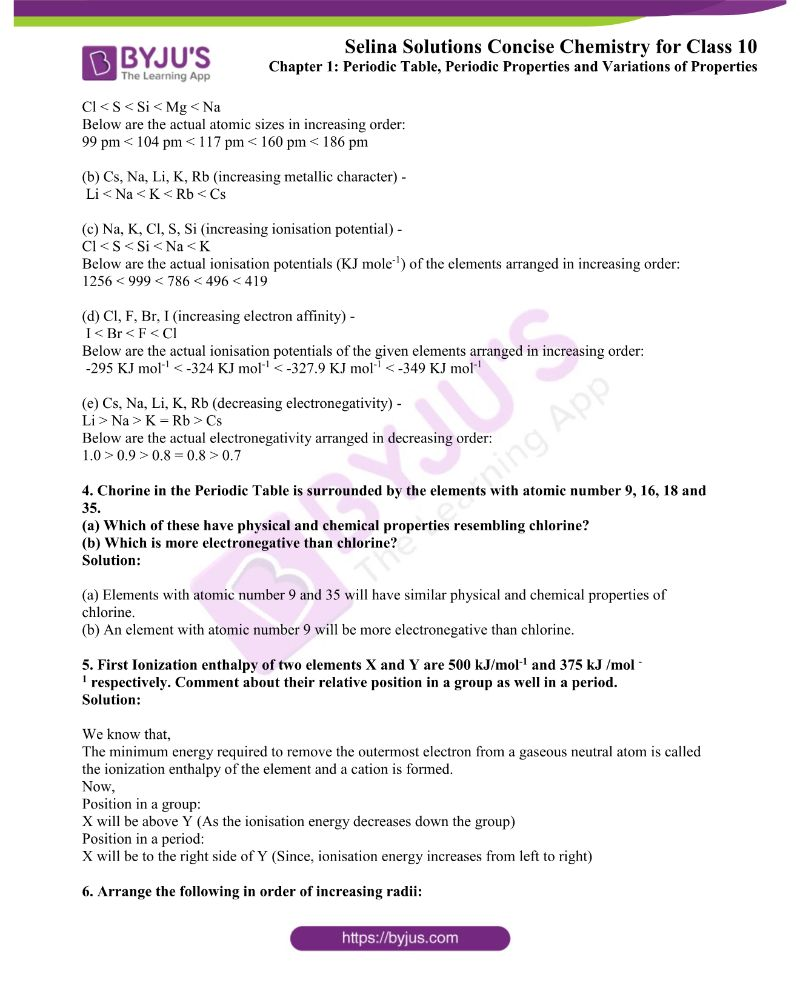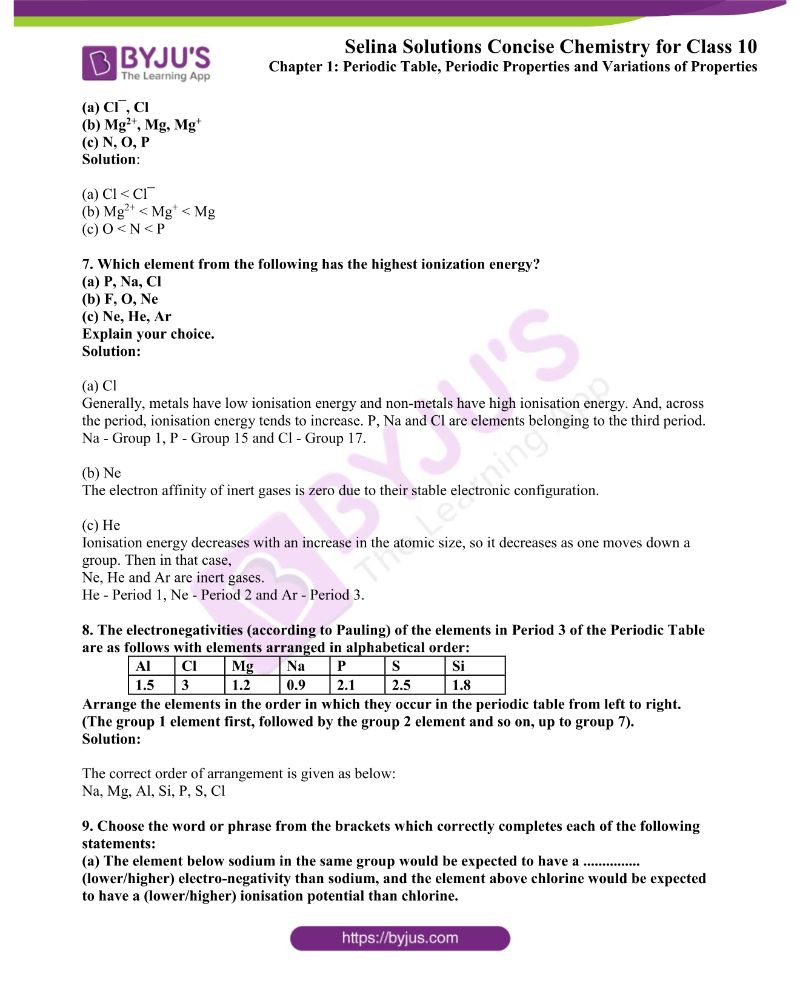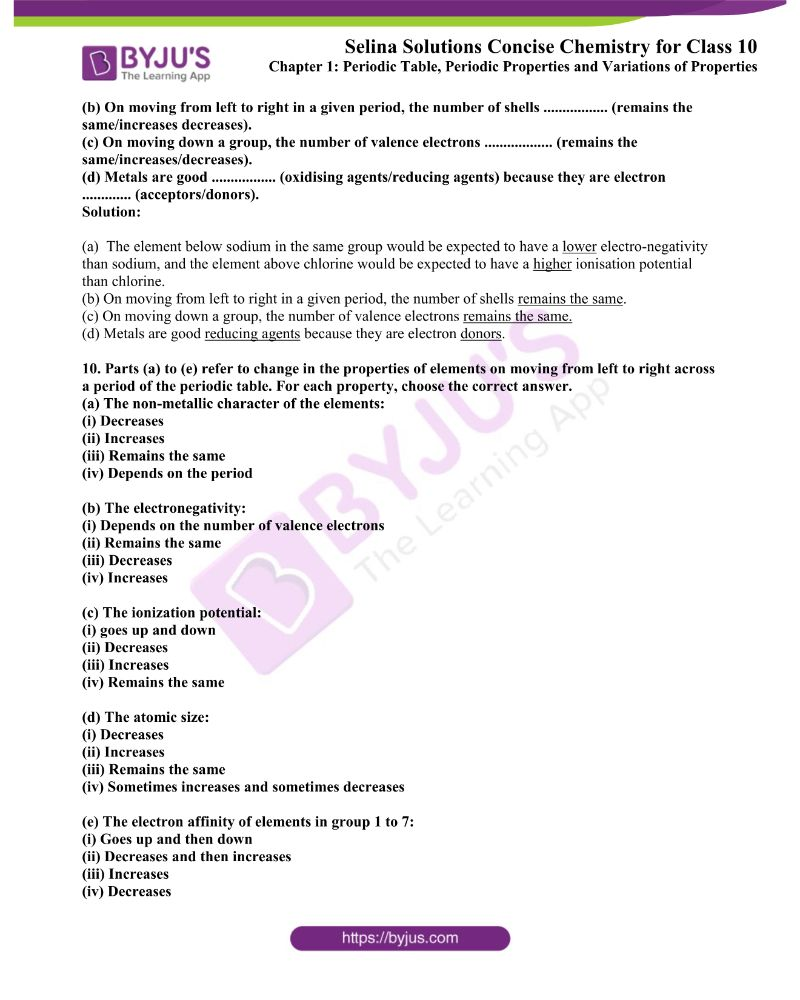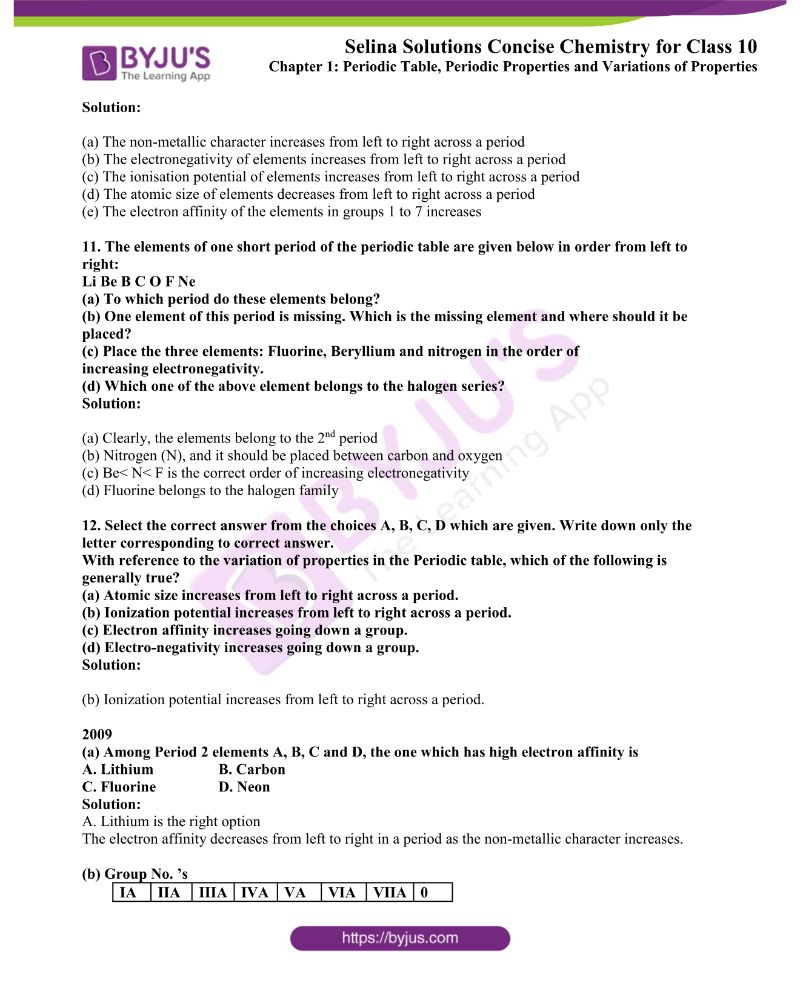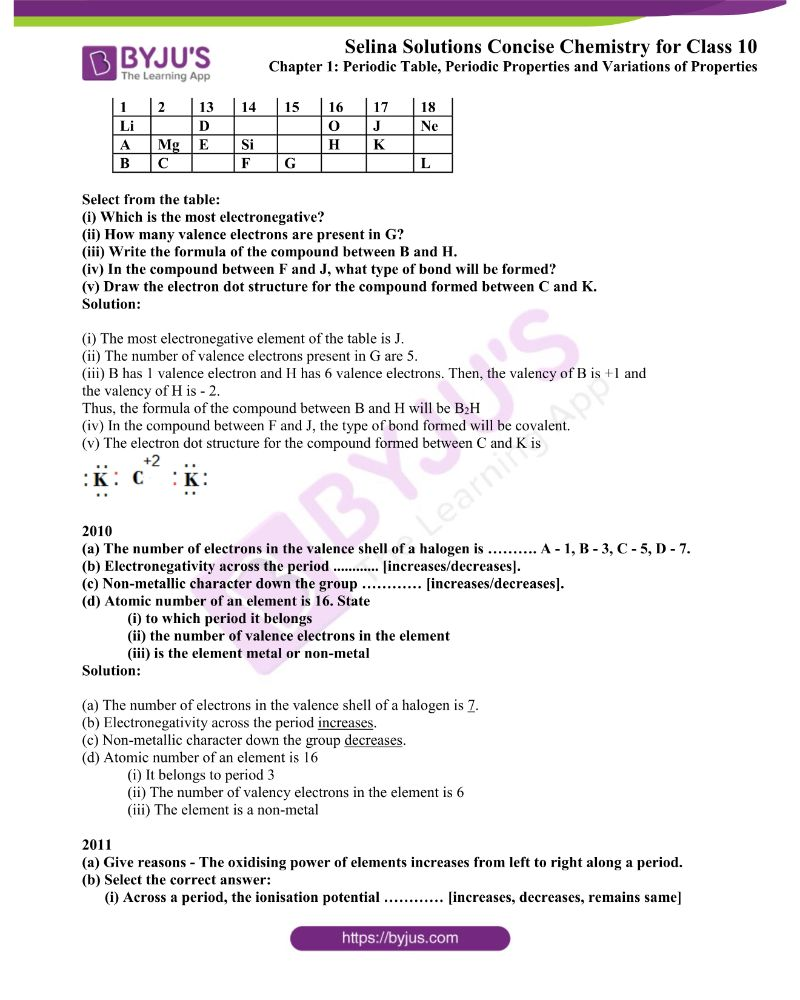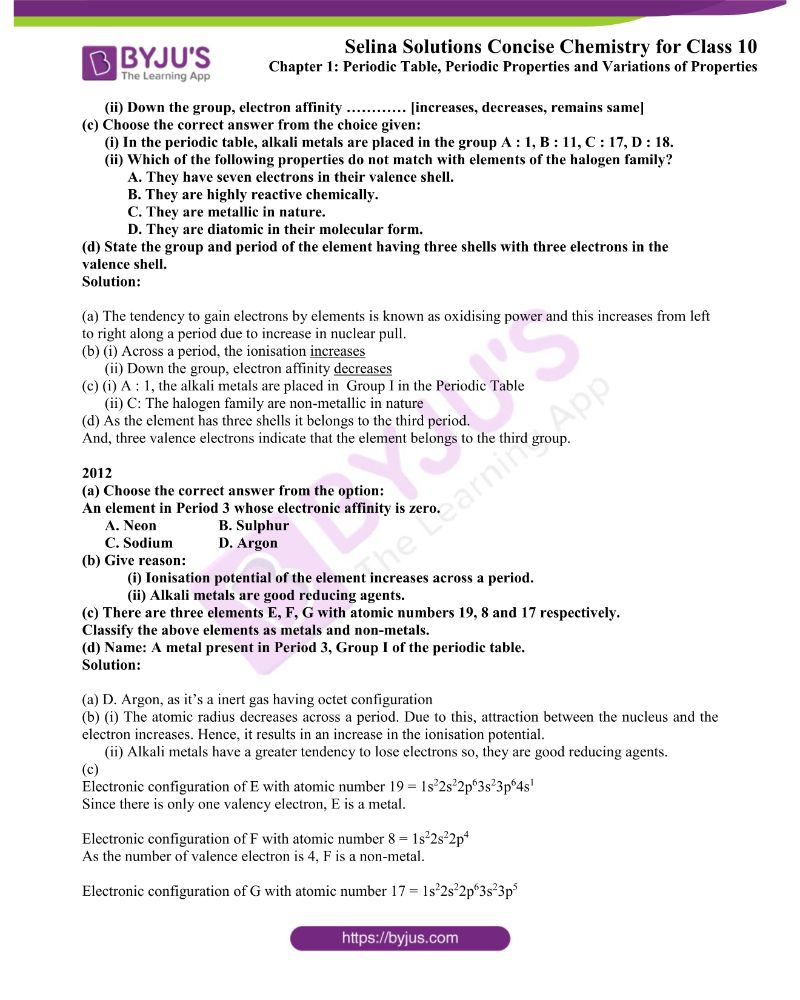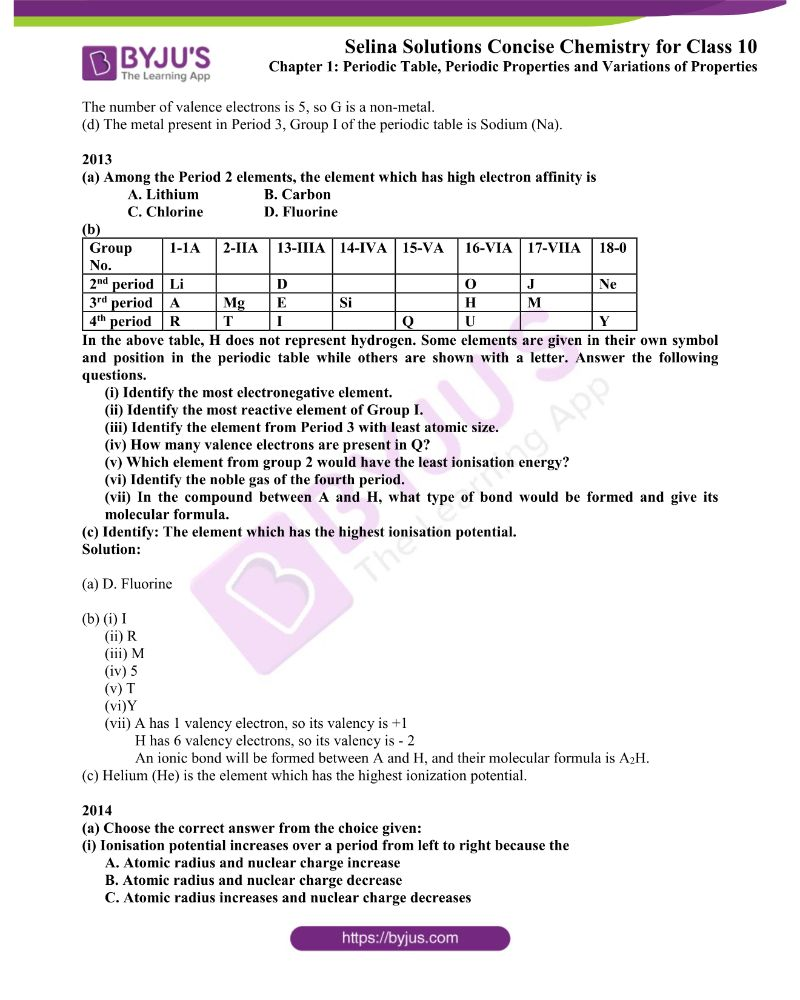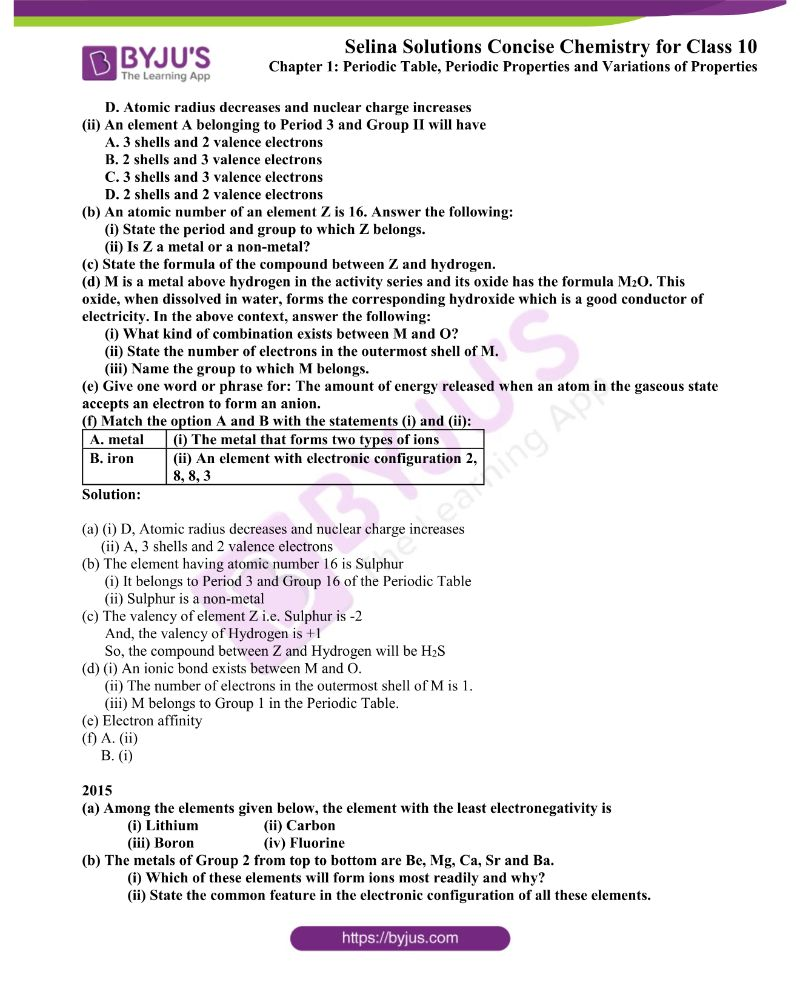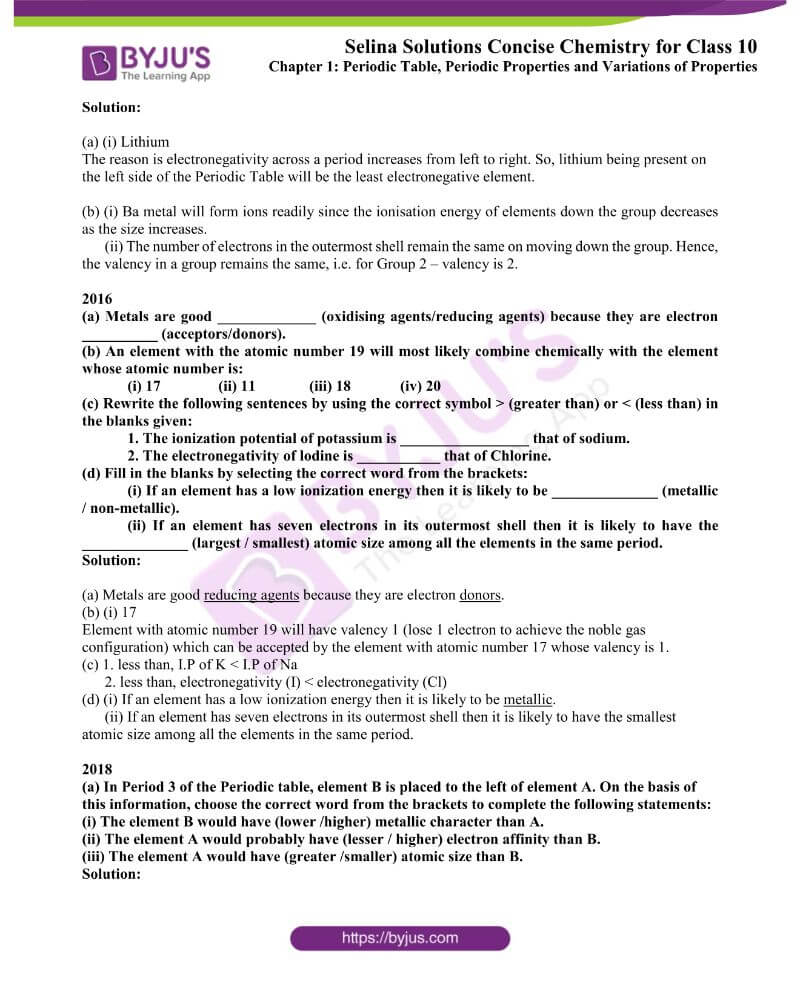### Access ICSE Selina Solutions for Class 10 Chemistry Chapter 1

Exercise-1 Page No: 17

1. Name

(a) An alkali metal in period 3 and halogen in period 2

(b) The noble gas with 3 shells

(c) The non-metals present in period 2 and metals in period 3.

(d) The element of period 3 with valency 4.

(e) The element in period 3 which does not form an oxide.

(f) The element of lower nuclear charge out of Be and Mg.

(g) Which has higher E.A. Fluorine or Neon?

(h) Which has maximum metallic character Na, Li or K?

Solution:

(a) Na and F

(b) Argon

(c) The non-metals present in period 2 are C, N, O and F, and the metals present in period 3 are Na, Mg and Al

(d) Silicon

(e) Argon

(f) Mg

(g) Fluorine

(h) K

2. Choose the most appropriate answer from

[SO2, SiO2, Al2O3, CO, MgO, Na2O]

(a) A covalent oxide of a metalloid.

(b) An oxide which when dissolved in water forms acid.

(c) A basic oxide.

(d) An amphoteric oxide.

Solution:

(a) A covalent oxide of a metalloid. – SiO2 (where, Si is a metalloid)

(b) An oxide which when dissolved in water forms acid. – SO2 (As, SO2 + H2O → H2SO3)

(c) A basic oxide. – Na2O (Na2O + H2O → 2NaOH)

(d) An amphoteric oxide. – Al2O3 (because it shows both acidic and basic properties)

3. Arrange the following as per instructions given in the brackets.

(a) Mg, Cl, Na, S, Si (increasing order of atomic size)

(b) Cs, Na, Li, K, Rb (increasing metallic character)

(c) Na, K, Cl, S, Si (increasing ionisation potential)

(d) Cl, F, Br, I (increasing electron affinity)

(e) Cs, Na, Li, K, Rb (decreasing electronegativity)

Solution:

(a) Mg, Cl, Na, S, Si (increasing order of atomic size) –

Cl < S < Si < Mg < Na

Below are the actual atomic sizes in increasing order:

99 pm < 104 pm < 117 pm < 160 pm < 186 pm

(b) Cs, Na, Li, K, Rb (increasing metallic character) –

Li < Na < K < Rb < Cs

(c) Na, K, Cl, S, Si (increasing ionisation potential) –

Cl < S < Si < Na < K

Below are the actual ionisation potentials (KJ mole-1) of the elements arranged in increasing order:

1256 < 999 < 786 < 496 < 419

(d) Cl, F, Br, I (increasing electron affinity) –

I < Br < F < Cl

Below are the actual ionisation potentials of the given elements arranged in increasing order:

-295 KJ mol-1 < -324 KJ mol-1 < -327.9 KJ mol-1 < -349 KJ mol-1

(e) Cs, Na, Li, K, Rb (decreasing electronegativity) –

Li > Na > K = Rb > Cs

Below are the actual electronegativity arranged in decreasing order:

1.0 > 0.9 > 0.8 = 0.8 > 0.7

4. Chorine in the Periodic Table is surrounded by the elements with atomic number 9, 16, 18 and 35.

(a) Which of these have physical and chemical properties resembling chlorine?

(b) Which is more electronegative than chlorine?

Solution:

(a) Elements with atomic number 9 and 35 will have similar physical and chemical properties of chlorine.

(b) An element with atomic number 9 will be more electronegative than chlorine.

5. First Ionization enthalpy of two elements X and Y are 500 kJ/mol-1 and 375 kJ /mol -1 respectively. Comment about their relative position in a group as well in a period.

Solution:

We know that,

The minimum energy required to remove the outermost electron from a gaseous neutral atom is called the ionization enthalpy of the element and a cation is formed.

Now,

Position in a group:

X will be above Y (As the ionisation energy decreases down the group)

Position in a period:

X will be to the right side of Y (Since ionisation energy increases from left to right)

6. Arrange the following in order of increasing radii:

(a) Cl¯, Cl

(b) Mg2+, Mg, Mg+

(c) N, O, P

Solution:

(a) Cl < Cl¯

(b) Mg2+ < Mg+ < Mg

(c) O < N < P

7. Which element from the following has the highest ionization energy?

(a) P, Na, Cl

(b) F, O, Ne

(c) Ne, He, Ar

Solution:

(a) Cl

Generally, metals have low ionisation energy and non-metals have high ionisation energy. And, across the period, ionisation energy tends to increase. P, Na and Cl are elements belonging to the third period.

Na – Group 1, P – Group 15 and Cl – Group 17.

(b) Ne

The electron affinity of inert gases is zero due to their stable electronic configuration.

(c) He

Ionisation energy decreases with an increase in the atomic size, so it decreases as one moves down a group. Then in that case,

Ne, He and Ar are inert gases.

He – Period 1, Ne – Period 2 and Ar – Period 3.

8. The electronegativities (according to Pauling) of the elements in Period 3 of the Periodic Table are as follows with elements arranged in alphabetical order:

 Al Cl Mg Na P S Si 1.5 3 1.2 0.9 2.1 2.5 1.8

Arrange the elements in the order in which they occur in the periodic table from left to right.

(The group 1 element first, followed by the group 2 element and so on, up to group 7).

Solution:

The correct order of arrangement is given as below:

Na, Mg, Al, Si, P, S, Cl

9. Choose the word or phrase from the brackets which correctly completes each of the following statements:

(a) The element below sodium in the same group would be expected to have a …………… (lower/higher) electro-negativity than sodium, and the element above chlorine would be expected to have a (lower/higher) ionisation potential than chlorine.

(b) On moving from left to right in a given period, the number of shells …………….. (remains the same/increases decreases).

(c) On moving down a group, the number of valence electrons ……………… (remains the same/increases/decreases).

(d) Metals are good …………….. (oxidising agents/reducing agents) because they are electron …………. (acceptors/donors).

Solution:

(a)  The element below sodium in the same group would be expected to have a lower electro-negativity than sodium, and the element above chlorine would be expected to have a higher ionisation potential than chlorine.

(b) On moving from left to right in a given period, the number of shells remains the same.

(c) On moving down a group, the number of valence electrons remains the same.

(d) Metals are good reducing agents because they are electron donors.

10. Parts (a) to (e) refer to change in the properties of elements on moving from left to right across a period of the periodic table. For each property, choose the correct answer.

(a) The non-metallic character of the elements:

(i) Decreases

(ii) Increases

(iii) Remains the same

(iv) Depends on the period

(b) The electronegativity:

(i) Depends on the number of valence electrons

(ii) Remains the same

(iii) Decreases

(iv) Increases

(c) The ionization potential:

(i) goes up and down

(ii) Decreases

(iii) Increases

(iv) Remains the same

(d) The atomic size:

(i) Decreases

(ii) Increases

(iii) Remains the same

(iv) Sometimes increases and sometimes decreases

(e) The electron affinity of elements in group 1 to 7:

(i) Goes up and then down

(ii) Decreases and then increases

(iii) Increases

(iv) Decreases

Solution:

(a) The non-metallic character increases from left to right across a period

(b) The electronegativity of elements increases from left to right across a period

(c) The ionisation potential of elements increases from left to right across a period

(d) The atomic size of elements decreases from left to right across a period

(e) The electron affinity of the elements in groups 1 to 7 increases

11. The elements of one short period of the periodic table are given below in order from left to right:

Li Be B C O F Ne

(a) To which period do these elements belong?

(b) One element of this period is missing. Which is the missing element and where should it be placed?

(c) Place the three elements: Fluorine, Beryllium and nitrogen in the order of increasing electronegativity.

(d) Which one of the above element belongs to the halogen series?

Solution:

(a) Clearly, the elements belong to the 2nd period

(b) Nitrogen (N), and it should be placed between carbon and oxygen

(c) Be< N< F is the correct order of increasing electronegativity

(d) Fluorine belongs to the halogen family

12. Select the correct answer from the choices A, B, C, D which are given. Write down only the letter corresponding to correct answer.

With reference to the variation of properties in the Periodic table, which of the following is generally true?

(a) Atomic size increases from left to right across a period.

(b) Ionization potential increases from left to right across a period.

(c) Electron affinity increases going down a group.

(d) Electro-negativity increases going down a group.

Solution:

(b) Ionization potential increases from left to right across a period.

2009

(a) Among Period 2 elements A, B, C and D, the one which has high electron affinity is

A. Lithium B. Carbon

C. Fluorine D. Neon

Solution:

A. Lithium is the right option

The electron affinity decreases from left to right in a period as the non-metallic character increases.

(b) Group No. ’s

 IA IIA IIIA IVA VA VIA VIIA 0 1 2 13 14 15 16 17 18 Li D O J Ne A Mg E Si H K B C F G L

Select from the table:

(i) Which is the most electronegative?

(ii) How many valence electrons are present in G?

(iii) Write the formula of the compound between B and H.

(iv) In the compound between F and J, what type of bond will be formed?

(v) Draw the electron dot structure for the compound formed between C and K.

Solution:

(i) The most electronegative element of the table is J.

(ii) The number of valence electrons present in G are 5.

(iii) B has 1 valence electron and H has 6 valence electrons. Then, the valency of B is +1 and the valency of H is – 2.

Thus, the formula of the compound between B and H will be B2H

(iv) In the compound between F and J, the type of bond formed will be covalent.

(v) The electron dot structure for the compound formed between C and K is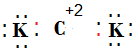2010

(a) The number of electrons in the valence shell of a halogen is ………. A – 1, B – 3, C – 5, D – 7.

(b) Electronegativity across the period ………… [increases/decreases].

(c) Non-metallic character down the group ………… [increases/decreases].

(d) Atomic number of an element is 16. State

(i) to which period it belongs

(ii) the number of valence electrons in the element

(iii) is the element metal or non-metal

Solution:

(a) The number of electrons in the valence shell of a halogen is 7.

(b) Electronegativity across the period increases.

(c) Non-metallic character down the group decreases.

(d) The atomic number of an element is 16

(i) It belongs to period 3

(ii) The number of valency electrons in the element is 6

(iii) The element is a non-metal

2011

(a) Give reasons – The oxidising power of elements increases from left to right along a period.

(i) Across a period, the ionisation potential ………… [increases, decreases, remains the same]

(ii) Down the group, electron affinity ………… [increases, decreases, remains the same]

(c) Choose the correct answer from the choice given:

(i) In the periodic table, alkali metals are placed in group A : 1, B : 11, C : 17, D : 18.

(ii) Which of the following properties do not match with elements of the halogen family?

A. They have seven electrons in their valence shell.

B. They are highly reactive chemically.

C. They are metallic in nature.

D. They are diatomic in their molecular form.

(d) State the group and period of the element having three shells with three electrons in the valence shell.

Solution:

(a) The tendency to gain electrons by elements is known as oxidising power and this increases from left to right along a period due to the increase in nuclear pull.

(b) (i) Across a period, the ionisation increases

(ii) Down the group, electron affinity decreases

(c) (i) A : 1, the alkali metals are placed in Group I in the Periodic Table

(ii) C: The halogen family are non-metallic in nature

(d) As the element has three shells it belongs to the third period.

And, three valence electrons indicate that the element belongs to the third group.

2012

(a) Choose the correct answer from the option:

An element in Period 3 whose electronic affinity is zero.

A. Neon B. Sulphur

C. Sodium D. Argon

(b) Give reason:

(i) The ionisation potential of the element increases across a period.

(ii) Alkali metals are good reducing agents.

(c) There are three elements E, F, G with atomic numbers 19, 8 and 17 respectively.

Classify the above elements as metals and non-metals.

(d) Name A metal present in Period 3, Group I of the periodic table.

Solution:

(a) D. Argon, as it’s an inert gas having octet configuration

(b) (i) The atomic radius decreases across a period. Due to this, the attraction between the nucleus and the electron increases. Hence, it results in an increase in the ionisation potential.

(ii) Alkali metals have a greater tendency to lose electrons so, they are good reducing agents.

(c)

Electronic configuration of E with atomic number 19 = 1s22s22p63s23p64s1

Since there is only one valency electron, E is a metal.

Electronic configuration of F with atomic number 8 = 1s22s22p4

As the number of valence electron is 4, F is a non-metal.

Electronic configuration of G with atomic number 17 = 1s22s22p63s23p5

The number of valence electrons is 5, so G is a non-metal.

(d) The metal present in Period 3, Group I of the periodic table is Sodium (Na).

2013

(a) Among the Period 2 elements, the element which has high electron affinity is

A. Lithium B. Carbon

C. Chlorine D. Fluorine

(b)

 Group No. 1-1A 2-IIA 13-IIIA 14-IVA 15-VA 16-VIA 17-VIIA 18-0 2nd period Li D O J Ne 3rd period A Mg E Si H M 4th period R T I Q U Y

In the above table, H does not represent hydrogen. Some elements are given in their own symbol and position in the periodic table while others are shown with a letter. Answer the following questions.

(i) Identify the most electronegative element.

(ii) Identify the most reactive element of Group I.

(iii) Identify the element from Period 3 with least atomic size.

(iv) How many valence electrons are present in Q?

(v) Which element from group 2 would have the least ionisation energy?

(vi) Identify the noble gas of the fourth period.

(vii) In the compound between A and H, what type of bond would be formed and give its molecular formula?

(c) Identify The element which has the highest ionisation potential.

Solution:

(a) D. Fluorine

(b) (i) I

(ii) R

(iii) M

(iv) 5

(v) T

(vi)Y

(vii) A has 1 valency electron, so its valency is +1

H has 6 valency electrons, so its valency is – 2

An ionic bond will be formed between A and H, and their molecular formula is A2H.

(c) Helium (He) is the element which has the highest ionization potential.

2014

(a) Choose the correct answer from the choice given:

(i) Ionisation potential increases over a period from left to right because the

A. Atomic radius and nuclear charge increase

B. Atomic radius and nuclear charge decrease

C. Atomic radius increases and nuclear charge decreases

D. Atomic radius decreases and nuclear charge increases

(ii) An element A belonging to Period 3 and Group II will have

A. 3 shells and 2 valence electrons

B. 2 shells and 3 valence electrons

C. 3 shells and 3 valence electrons

D. 2 shells and 2 valence electrons

(b) An atomic number of an element Z is 16. Answer the following:

(i) State the period and group to which Z belongs.

(ii) Is Z a metal or a non-metal?

(c) State the formula of the compound between Z and hydrogen.

(d) M is a metal above hydrogen in the activity series and its oxide has the formula M2O. This oxide, when dissolved in water, forms the corresponding hydroxide which is a good conductor of electricity. In the above context, answer the following:

(i) What kind of combination exists between M and O?

(ii) State the number of electrons in the outermost shell of M.

(iii) Name the group to which M belongs.

(e) Give one word or phrase for: The amount of energy released when an atom in the gaseous state accepts an electron to form an anion.

(f) Match the option A and B with the statements (i) and (ii):

 A. metal (i) The metal that forms two types of ions B. iron (ii) An element with electronic configuration 2, 8, 8, 3

Solution:

(a) (i) D, Atomic radius decreases and nuclear charge increases

(ii) A, 3 shells and 2 valence electrons

(b) The element having atomic number 16 is Sulphur

(i) It belongs to Period 3 and Group 16 of the Periodic Table

(ii) Sulphur is a non-metal

(c) The valency of element Z i.e. Sulphur is -2

And, the valency of Hydrogen is +1

So, the compound between Z and Hydrogen will be H2S

(d) (i) An ionic bond exists between M and O.

(ii) The number of electrons in the outermost shell of M is 1.

(iii) M belongs to Group 1 in the Periodic Table.

(e) Electron affinity

(f) A. (ii)

B. (i)

2015

(a) Among the elements given below, the element with the least electronegativity is

(i) Lithium (ii) Carbon

(iii) Boron (iv) Fluorine

(b) The metals of Group 2 from top to bottom are Be, Mg, Ca, Sr and Ba.

(i) Which of these elements will form ions most readily and why?

(ii) State the common feature in the electronic configuration of all these elements.

Solution:

(a) (i) Lithium

The reason is electronegativity across a period increases from left to right. So, lithium being present on the left side of the Periodic Table will be the least electronegative element.

(b) (i) Ba metal will form ions readily since the ionisation energy of elements down the group decreases as the size increases.

(ii) The number of electrons in the outermost shell remains the same on moving down the group. Hence, the valency in a group remains the same, i.e. for Group 2 – valency is 2.

2016

(a) Metals are good _____________ (oxidising agents/reducing agents) because they are electron __________ (acceptors/donors).

(b) An element with the atomic number 19 will most likely combine chemically with the element whose atomic number is:

(i) 17 (ii) 11 (iii) 18 (iv) 20

(c) Rewrite the following sentences by using the correct symbol > (greater than) or < (less than) in the blanks given:

1. The ionization potential of potassium is _________________ that of sodium.

2. The electronegativity of lodine is ___________ that of Chlorine.

(d) Fill in the blanks by selecting the correct word from the brackets:

(i) If an element has a low ionization energy then it is likely to be ______________ (metallic / non-metallic).

(ii) If an element has seven electrons in its outermost shell then it is likely to have the ______________ (largest / smallest) atomic size among all the elements in the same period.

Solution:

(a) Metals are good reducing agents because they are electron donors.

(b) (i) 17

Element with atomic number 19 will have valency 1 (lose 1 electron to achieve the noble gas configuration) which can be accepted by the element with atomic number 17 whose valency is 1.

(c) 1. less than, I.P of K < I.P of Na

2. less than, electronegativity (I) < electronegativity (Cl)

(d) (i) If an element has low ionization energy then it is likely to be metallic.

(ii) If an element has seven electrons in its outermost shell then it is likely to have the smallest atomic size among all the elements in the same period.

2018

(a) In Period 3 of the Periodic table, element B is placed to the left of element A. On the basis of this information, choose the correct word from the brackets to complete the following statements:

(i) The element B would have (lower /higher) metallic character than A.

(ii) The element A would probably have (lesser / higher) electron affinity than B.

(iii) The element A would have (greater /smaller) atomic size than B.

Solution:

(i) The element B would have a higher metallic character than A.

(ii) The element A would probably have higher electron affinity than B.

(iii) The element A would have a smaller atomic size than B.

The given solutions are as per the 2019-20 Concise Selina textbook. The Selina Solutions for the academic year 2023-24 will be updated soon.